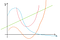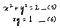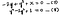# Equations

One of the first things we learn is numbers. Then they learn to perform operations like addition, subtraction, etc. on those numbers. Then comes the first level of abstraction; replace numbers with symbols. And this is where the world of equations starts. The simplest equation is something like:

x = 0 ______(1)

If I ask you what is ‘x’ given the above equation, it’s so trivial it’s silly. Similarly, if I add another equation:

y=3 _______(2)

and then ask you what are x and y, it is still trivial. The two equations above are linear equations. This is because the set of points that satisfy them lie on a straight line. Now, if we multiply both sides of equation (1) by a constant (say 5), the equation is still valid. If we call this constant a, we get: a x = 0. The number of possible equations we can get by changing a form an infinite set. This infinite set of equations is called an ideal. Similarly, multiplying the second one by b results in b y = 3 b. We get another ideal. And if we add these, we get a x + by = 3 b. This forms a third ideal of linear equations. An example of two other equations from this ideal might be (a=1, b=2) and (a=-1, b=2/3):

x+2 y = 6 ______(3)

3 x+2 y=2 ______(4)

Now if I gave you these two equations from the start, you would have had to spend slightly more mental cycles to get the solution. So, in a way, equations (1) and (2) are “better” representatives of their ideal than (3) and (4). This is because each of them involves just one variable. There is an algorithm for converting any system of linear equations to a form similar to equations (1) and (2) where we can just read off the solution. This algorithm is called Gaussian elimination and involves mashing the equations together with the goal of eliminating the variables one at a time.Solving systems of linear equations

# Polynomial equations

With linear equations, we are restricted to equations that draw out straight lines when plotted. This is another way of saying they can only take the form (if the variables are x and y): a x + b y = c. This means we can have the green line, but not the orange, red or blue ones in the figure below when we describe our equations.Only the green line is possible with linear equations

However, if we allow terms that have the squares of x and y, we can get the red curve; if we allow cubes, we can get the orange or blue ones while if we allow arbitrary powers of these two variables, we can get any curve one might squiggle on the graph. Due to the versatility of polynomial equations, having a general algorithm for solving them would be very powerful. Gaussian elimination should be a special case of this algorithm, when all the equations happen to be linear. Such an algorithm exists and is called “Buchberger’s algorithm”.

# Polynomial Ideals

Like we described the linear ideals for systems of liner equations (set of all equations we can get from linear combinations of the given equations), we can similarly define ideals for a system of polynomial equations. Then just like equations (1) and (2) for the linear ideal, we can reason about the best polynomial representatives of the polynomial ideal. For a concrete example, consider the system of quadratic equations:System of polynomial equations

It’s not too hard to work out that x=1 and y=1 satisfy both these equations. Like with the linear equations, we can multiply these with arbitrary constants and add them together to get other equations in their ideal. However, because these are polynomial equations, we can also multiply them with other polynomials and the resulting expression we get will still be a polynomial. But just like equations (1) and (2) were “special” equations for their ideal, we’re interested in the two special equations that best represent this ideal. For the linear case, in Gaussian elimination, we took the approach of trying to eliminate one of the variables. So, we were implicitly saying we value the variable we were eliminating less and wanted it out of the equations. You’ll observe that the polynomial equation is composed of elements called “monomials”. Things like x², y², xy, x³y, etc. Just like we decided some preference order for variables in the case of linear equations, we’ll need to define some preference order for the monomial terms that make up the polynomial equations. Then, we can try and devise an algorithm that tries to get rid of the terms that are lower priority from the polynomial equations. If our priority order happens to de-prioritize any monomials that contain one of the variables, we’ll end up eliminating that variable. So, we’ll end up with an equation in only one of the variables, which we can then hopefully solve. There are multiple ways to specify such ordering schemes for the monomials. The most common is “lexicographic” where we simply treat the powers of the variables as digits. For example, x²y corresponds to the number 21 (power of x; power of y) and xy² corresponds to the number 12. So, x²y will be prioritized higher than xy². Once we describe this order, the algorithm knows what monomials (and hence variables) to eliminate and gives us equations that don’t involve them (hence making them relatively easier to solve, just like in Gaussian elimination). For equations (5) and (6) for example, the algorithm produces the following two equations:Groebner basis for system of polynomial equations (5) and (6)

Equation (8) involves only the variable y and can be solved quite easily. It can then be substituted into equation (7) and this will allow us to solve for the variable, x. We can say these two equations form a new “basis” for the ideal (since all other equations in the ideal including (5) and (6) can be obtained from these two by multiplying them with appropriate polynomials and adding). This special basis that facilitates finding solutions for the equations is called the “Groebner basis” and the algorithm we’ve been referencing (“Buchberger’s algorithm”) helps reduce any system of polynomial equations to this Groebner basis. We’ve been a little hand-wavy about this algorithm and not gone into the specifics of how it works. This is described in section 2.7 of the book “Ideals, Varieties and Algorithms” by Cox, Little and O’Shea.

# Software Libraries

There are various software libraries that implement Buchberger’s algorithm. My favorite one is sympy (symbolic computation in python). Here is some simple sample code that shows how to define some symbolic variables, polynomials as functions of them and finally compute the Groebner basis.

You can see that the last polynomial equation in the Groebner basis involves only z. This can be solved numerically and the result substituted into the penultimate equation to get y and finally the values of y and z substituted into the first one to get x.

Of course, the library also provides a way to get the solution directly (but good to know what’s happening behind the covers).

Even though these libraries are available, it is quite satisfying to implement the algorithms from scratch. We cover one such implementation in C# in the next section.

# Implementation from scratch

It is quite satisfying that this whole theory of polynomials, their fields, etc. lends itself quite readily to the abstraction abilities of object oriented programming. The various objects — monomials, polynomials, etc. are surprisingly conducive to the constructs of object oriented programming. Each of them can be represented quite well as classes, with constructs building on top of each other. Also, the book by Cox Little and O’Shea builds up very nicely to Buchberger’s algorithm and it’s a lot of fun to actually implement it and see it in action. I chose C# as the language of implementation and open sourced the code (see here). The various classes that are a part of this solution form a hierarchical structure:

`PolynomialBasis||_Polynomial  |  |_Monomial`

What this means is that a collection of monomials form a polynomial and a collection of polynomials form a polynomial basis.

The most basic class is the monomial class and is backed up simply by an array. For example, x²y³z corresponds to the array [2,3,1]. As mentioned previously, we need to settle on an ordering scheme where given two monomials, we can clearly tell which one should get a higher preference. This lends itself beautifully to the concept of comparators in C#, where we define a method that returns 1 if the first object passed to it is “preferable”, -1 if the second one is and 0 if they should get the same preference. Then, any kind of sorting or other algorithms which work on preference order of the Monomial objects will just use your comparator to decide the preferences.

Since a polynomial basis is simply a set of polynomials, I used a HashSet to store the polynomials. However, when we think of monomials in a polynomial, the order matters. Also, we need to store not just the monomials but also their coefficients. So, I used a SortedDictionary of monomials to back up the polynomial object.

The code starts with the building blocks like LCM (Least Common Multiple), polynomial division, etc. and builds up to Groebner basis. Some applications of Groebner basis are also provided. You can execute the code via Program.cs. In the comments and documentation for the code, “CLO” referes to the CLO book.

Let’s see an example of how to use this code to solve systems of polynomial equations. A new Polynomial basis object can be instantiated through strings which contain polynomial equations in human readable form -

And then, the Buchbergers algorithm can be called to simplify the basis to a more natural Groebner basis. Then, we use the pretty print function to show this new basis.

This will execute the Buchberger’s algorithm and produce output in the following format -

`Polynomial - 0 + 0.67-0.78 z - 0.22 z^2 - 0.11 z^3 +  xPolynomial - 1-2.67 - 0.22 z + 0.22 z^2 + 0.11 z^3 +  yPolynomial - 2-18 - 12z + 13z^2 + 5z^3 +  z^4`

Notice that the last polynomial involves only z. We can use it to find possible solutions for this variable. The second to last one then, contains only z and y. So, the values of z calculated from the previous equation can be substituted to get possible values of y. Similarly, the first equation can be used to calculate x.

Written by

## Rohit Pandey

#### I’m a Data Scientist working for Microsoft Azure. I love to blog about Math topics.

Welcome to a place where words matter. On Medium, smart voices and original ideas take center stage - with no ads in sight. Watch
Follow all the topics you care about, and we’ll deliver the best stories for you to your homepage and inbox. Explore
Get unlimited access to the best stories on Medium — and support writers while you’re at it. Just \$5/month. Upgrade